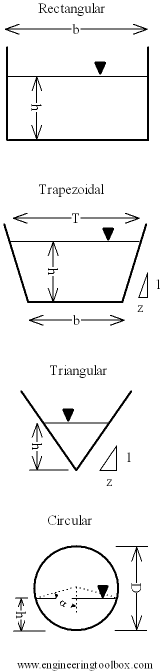Engineering ToolBox - Resources, Tools and Basic Information for Engineering and Design of Technical Applications!

# Flow Section Channels - Geometric Relationships

## Geometric relationships like area, wetted perimeter and hydraulic diameter.

Area, wetted perimeter and hydraulic diameter for geometric sections like

• rectangular channels
• trapezoidal channels
• triangular channels
• circular channels### Rectangular Channel

#### Flow Area

Flow area of a rectangular channel:

A = b h                               (1)

where

A = flow area (m2, in2)

b = width of channel (m, in)

h = height of flow (m, in)

#### Wetted Perimeter

Wetted perimeter of a rectangular channel:

P = b + 2 h                         (1b)

where

P = wetted perimeter (m, in)

Hydraulic radius of a rectangular channel:

Rh = b h / (b + 2 h)                   (1c)

where

Rh = hydraulic radius (m, in)

### Trapezoidal Channel

#### Flow Area

Flow area of a trapezoidal channel:

A = h (b + T) / 2                      (2)

#### Wetted Perimeter

Wetted perimeter of a trapezoidal channel:

P = b + 2 (((T - b) / 2)2 + h2)1/2                       (2b)

Hydraulic radius of a trapezoidal channel:

Rh = (h (b + T) / 2) / (b + 2 (((T - b) / 2)2 + h2)1/2)                       (2c)

### Triangular Channel

#### Flow Area

Flow area of a triangular channel:

A = z h2                     (3)

where

z = see figure above (m, in)

#### Wetted Perimeter

Wetted perimeter of a triangular channel:

P = 2 h (1 + z2)1/2                      (3b)

Hydraulic radius of a triangular channel:

Rh = z h / 2 (1 + z2)1/2                      (3c)

### Circular Channel

#### Flow Area

Flow area of a circular channel:

A = D2/4 (α - sin(2 α)/2)                      (4)

where

D = diameter of channel

α = cos-1(1 - h/r)

#### Wetted Perimeter

Wetted perimeter of a circular channel:

P = α D                      (4b)

Hydraulic radius of a circular channel:

Rh = D/4 [1 - sin(2 α) / (2 α)]                     (4c)

## Related Topics

• Fluid Mechanics - The study of fluids - liquids and gases. Involving velocity, pressure, density and temperature as functions of space and time.

## Engineering ToolBox - SketchUp Extension - Online 3D modeling!

Add standard and customized parametric components - like flange beams, lumbers, piping, stairs and more - to your Sketchup model with the Engineering ToolBox - SketchUp Extension - enabled for use with the amazing, fun and free SketchUp Make and SketchUp Pro .Add the Engineering ToolBox extension to your SketchUp from the SketchUp Pro Sketchup Extension Warehouse!

Translate

## Privacy

We don't collect information from our users. Only emails and answers are saved in our archive. Cookies are only used in the browser to improve user experience.

Some of our calculators and applications let you save application data to your local computer. These applications will - due to browser restrictions - send data between your browser and our server. We don't save this data.

## Citation

• Engineering ToolBox, (2005). Flow Section Channels - Geometric Relationships. [online] Available at: https://www.engineeringtoolbox.com/flow-section-channels-d_965.html [Accessed Day Mo. Year].

Modify access date.

. .

#### Scientific Online Calculator3 10